# Graphs using ggplot2 package in R: Compare qplot() vs ggplot()

You can downloed Rmd solution file as well as the tuition.csv dataset.

The aim of the following tasks is to use analytic graph tools in R to investigate the relationship between tuition and other variables. The dataset used is called tuition.csv and it has 1,283 records of information about school tuition.

mdata <- read.csv("tuition.csv")

## Part 1: qplot() from ggplot2 package

library(ggplot2)
1. Make sure the data type of public.private is factor. Rename the level name to {public, private} instead of {0; 1} by levels(mdata$public.private) <- c(“public”, “private”). class(mdata$public.private)
##  "integer"
mdata$public.private <- as.factor(mdata$public.private)
levels(mdata$public.private) <- c("public", "private") 1. Rename the column public.private as “School Type” by colnames() function. colnames(mdata) <- "School Type" 1. Give a histogram displaying the frequency counts of the public school tuition and private school tuition together. HINT: Call qplot() with 3 arguments. First specify the variable for which you want the frequency count, in this case tuition, then specify the data, and finally, the aesthetic, fill, set equal to School Type. qplot(tuition, data = mdata, fill = School Type)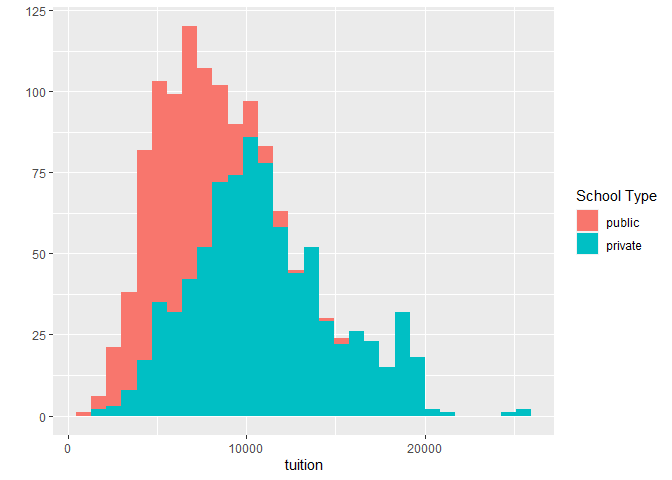1. Redo part (c) by making 2 smaller individual histograms of each subset. List them column-wise in one plot. HINT: With one call to qplot(), work on argument facet. qplot(tuition, data = mdata, facets = School Type ~ .)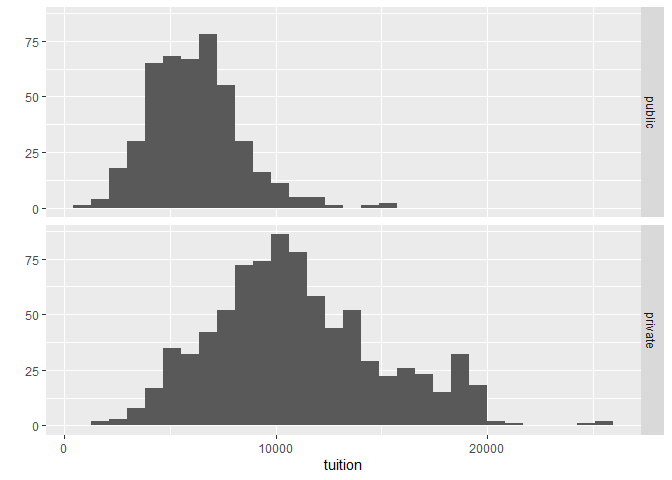1. Modify the plot in part (d) as following: title is “Tuition Between the Public and Private School”; y label is “US Dollar”; x label is “The Number of Schools”. qplot(tuition, data = mdata, facets = School Type ~ ., main = "Tuition Between the Public and Private School", xlab = "US Dollar", ylab = "The Number of Schools")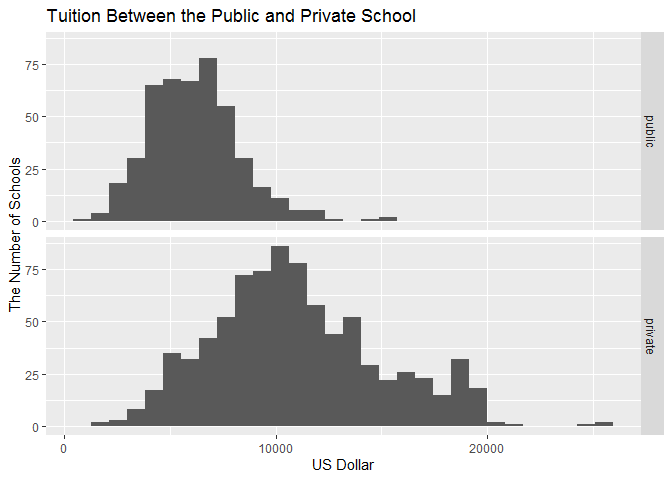1. Give two boxplots listing column-wise in one plot to explain the relationship between tuition and School Type. HINT: With one call to qplot(), work on argument geom to get boxplot. Unlike the drawing histogram by qplot(), the first three arguments of it could be: • First specify the variable by which you’ll split the data. • Then specify the variable which you want to examine. • The third argument is data source. qplot(School Type, tuition, data = mdata, geom = "boxplot")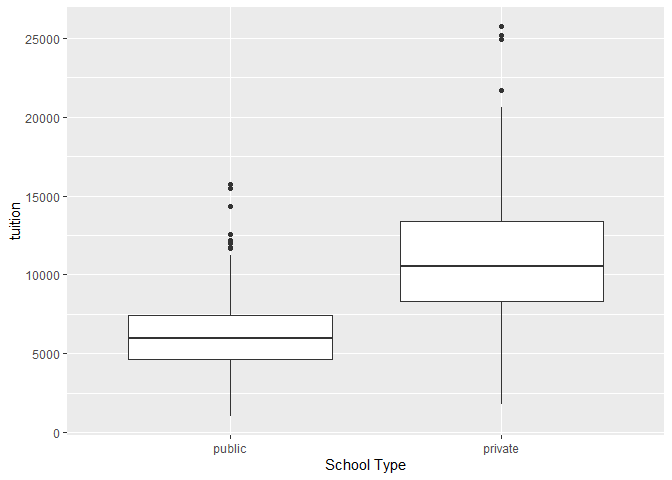1. Redo part (f) by white background color, font size is 14 and font family equals to “Times”. qplot(School Type, tuition, data = mdata, geom = "boxplot") + theme_bw() + theme(axis.text = element_text(size = 14, family = "Times"), axis.title = element_text(size = 14, family = "Times"))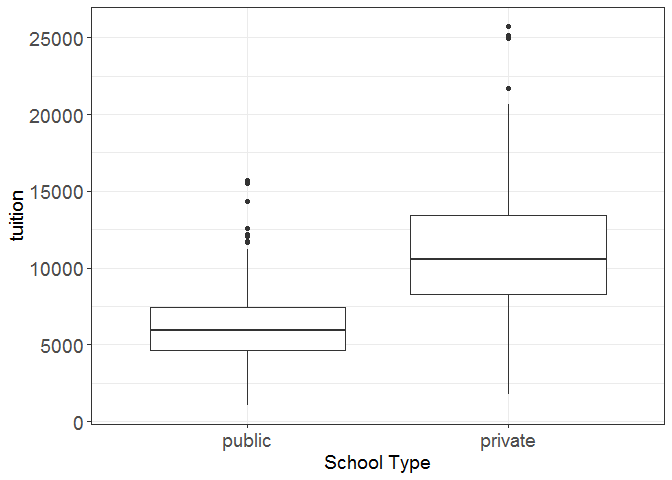## Part 2: ggplot() from ggplot2 package 1. Redo the whole Part 1 by ggplot() function. Histogram displaying the frequency counts of the public school tuition and private school tuition together. ggplot(mdata, aes(tuition)) + geom_histogram(aes(fill = School Type))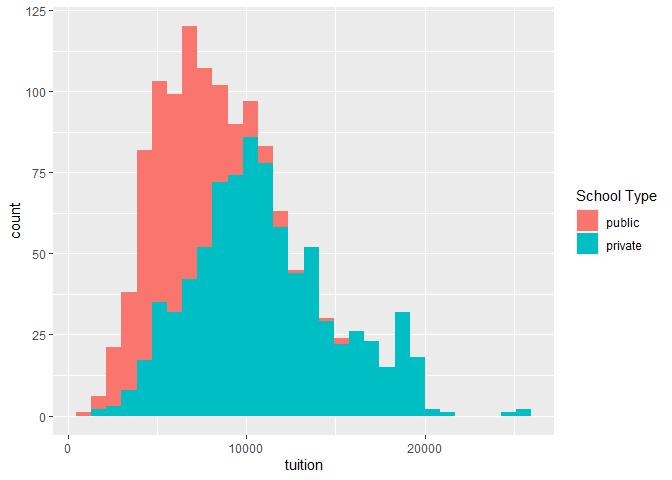2 smaller individual histograms of each subset. ggplot(mdata, aes(tuition)) + geom_histogram() + facet_grid(School Type ~ .)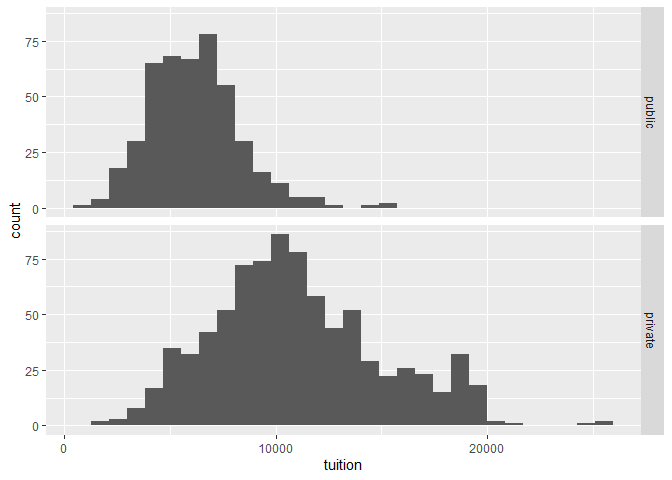Add title and axes labels. ggplot(mdata, aes(tuition)) + geom_histogram() + facet_grid(School Type ~ .) + labs(title = "Tuition Between the Public and Private School", x = "US Dollar", y = "The Number of Schools")Two boxplots listing column-wise in one plot to explain the relationship between tuition and School Type. ggplot(mdata, aes(School Type, tuition)) + geom_boxplot()Change background color and font. ggplot(mdata, aes(School Type, tuition)) + geom_boxplot() + theme_bw() + theme(axis.text = element_text(size = 14, family = "Times"), axis.title = element_text(size = 14, family = "Times"))1. Create a scatter plot describing the correlation between pct_phd and tuition, respectively for public and private school. The points for public school is red solid triangle, while that for private school is blue snowflakes. Add two linear regression lines corresponding to different kind of school. Specify appropriate title, labels and legends to the plot. ggplot(mdata, aes(pct_phd, tuition)) + geom_point(aes(col = School Type, shape = School Type)) + # point colors scale_colour_manual(values = c("red", "blue")) + # point shapes scale_shape_manual(values = c(17, 8)) + # add linear regression lines geom_smooth(aes(col = School Type), method = "lm", se = FALSE) + # specify title and labels labs(title = "Tuition vs. Faculty PhD degrees \nfor Public and Private Schools", x = "Percent of faculty with PhD's.", y = "Tuition, US Dollar")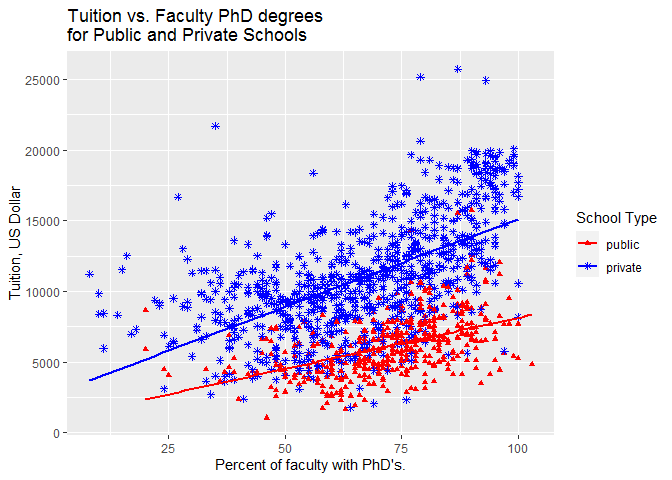1. In order to study the relationship between tuition, types of school, faculty compensation and faculty PhD degrees, • Categorize pct_phd Percent of faculty with Ph.D.’s by dividing it into 10 equal groups (cut pct_phd at the deciles). Create a new factor variable pct_phd2dec into data frame mdata. mdata$pct_phd2dec <- cut(mdata$pct_phd, quantile(mdata$pct_phd, (0:10)/10, na.rm = TRUE),
include.lowest = TRUE)
• Apply ggplot() with the updated mdata. The x is fac_comp and y is tuition.
g <- ggplot(mdata, aes(fac_comp, tuition))
• Provide the scatter plots of x and y based on the combination of School Type and pct_phd2dec. Give your comments on the plot and summarize the relationship between tuition, types of school, faculty compensation and faculty PhD degrees.
g +
geom_point() +
facet_grid(School Type ~ pct_phd2dec, margins = TRUE) +
labs(x = "Faculty Compensation, US Dollar",
y = "Tuition, US Dollar")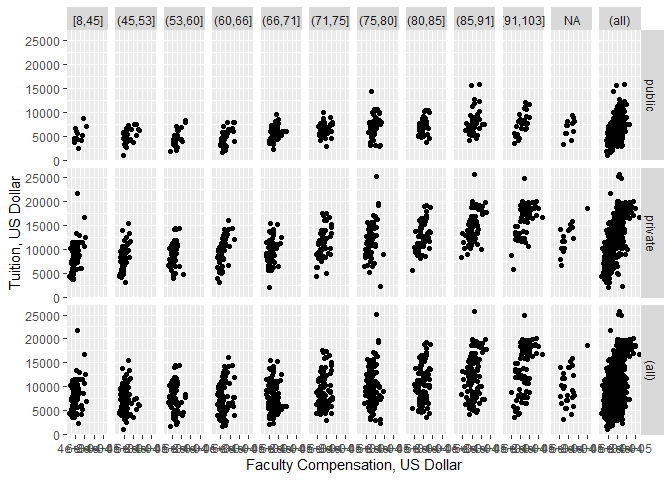The scatterplots show that for both public and private schools, as the average faculty compensation increases, the tuition also increases. Also, it seems that the average faculty compensation is higher for schools where higher percentage of faculty has PhD degrees.

The scatterplots also show that tuition for private schools tends to be higher than tuition for public schools. However, for both public and private schools the tuition seems to increase as the percentage of faculty with PhD’s increases, although this trend is more pronounced for private schools.